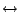Next: The source and the Up: transIT: A Prolog tool Previous: Introduction

# A glimpse of the translation process

As mentioned the translation process is carried out by means of a Prolog program. Such program takes as input a formula of a specific source language (see Section 2) and performs a sequence of rewriting transformations. The following sequence of phases are usually performed (some of them might be skipped, for instance intuitionistic double-negation is not removed):

Lexical and syntactical analyses.
Depending on the specific language, this phase accepts a formula only if its constructs belong to the language and the formula is well-formed (i.e., syntactically correct). The syntax-directed translation implemented through this stage is described by an (attributed) definite clause grammar. Hence, any extension to further logics can be achieved by simply adding a suitable set of grammar rules which characterize the (new) well-formed formulas.

The outcome of this stage consists in an intermediate representation of the abstract syntax tree (AST) of the input formula.

Generation of an internal representation.
By means of a rewriting process (acting in a bottom-up recursive fashion) the outcome of the preceding phase is turned into an internal representation of the AST which is independent of the source language.
Reduction to primitive constructs.
In this phase the formula is rewritten in terms of a restricted set of constructs and connectives, to be intended as ``primitive''. For instance, biimplicationis rewritten in terms of implication, and so on. Notice that some of the rewritings could be inhibited, because unsound with respect to the specific logic at hand. The aim of this transformation consists in simplifying the next phase.
Propositional simplifications.
Through this phase the internal representation of the formula is simplified by applying a number of propositional simplifications mainly aimed at reducing the size of the formula (for instance, by eliminating tautological sub-formulas, double negations, and so on).
Relational translation.
This is the main step of the translation process: the internal representation of the given formula is translated into the calculus of dyadic relations. The kind of rewriting rules employed may depend on the source language of the input formula (see Section 2). The outcome of this phase is a relational term.
Relational simplifications.
The translation process ends by applying a series of relational simplifications on the relational term produced by the preceding step. The simplest among these rewritings take care of the idempotence, absorption or involution properties of (some of) the relational constructs. The process can be easily extended to perform more complex simplification.
As a matter of fact, further phases could be added, for instance in order to apply semantical transformations to the relational term, possibly with respect to a set of axiomatic assumption characterizing a particular class of relational structures as target framework.Next: The source and the Up: transIT: A Prolog tool Previous: Introduction
Last update: 02-08-2005 by andy